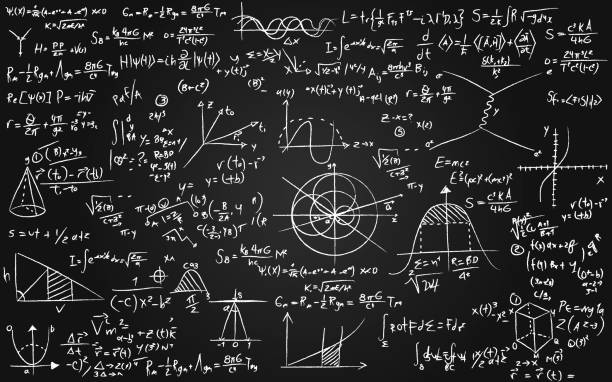First of all what is a quadratic equation?

A quadratic equation is an equation that you can write it in the form of $ax^2+bx+c=0$. For example, $x^2+x+5=0$. Next, how to solve this equation.

There are some methods to solve this type of quadratic equation. One of the main methods is to use the quadratic formula.Math equations written on a blackboard – mathematics and science concepts

The quadratic formula: $\dfrac{-b\pm \sqrt{b^2-4ac}}{2a}$ gives us the solutions. There are some important points:

• If $b^2-4ac$ is negative, there is no solution.
• If $b^2-4ac =0$, we have only one solution.
• If $b^2-4ac$ is positive, we have two solutions.
• The sum of solutions is: $\dfrac{-b}{a}$
• The product of solutions is : $\dfrac{c}{a}$.

If you need help to understand the quadratic formula, contact us.

Read More: Linear Equations